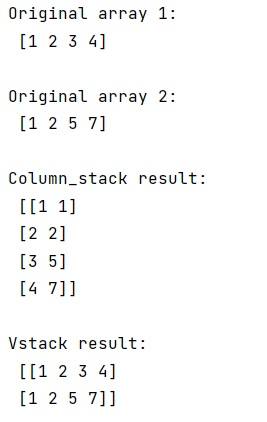# NumPy vstack vs. column_stack

Learn about the difference between numpy vstack() and column_stack() methods.
Submitted by Pranit Sharma, on January 25, 2023

NumPy is an abbreviated form of Numerical Python. It is used for different types of scientific operations in python. Numpy is a vast library in python which is used for almost every kind of scientific or mathematical operation. It is itself an array which is a collection of various methods and functions for processing the arrays.

## NumPy's vstack() Vs. column_stack()

The key difference between these two methods is that column_stack() stacks horizontally and vstack() vertically.

When we use column_stack() to append a column we want, we need to convert it from a 1-dimensional array to a 2-dimensional column because a 1d array is normally interpreted as a vector-row in a 2d context in NumPy.

Let us understand with the help of an example,

## Python code to demonstrate the example of numpy vstack() and column_stack() methods

```# Import numpy
import numpy as np

# Creating two numpy arrays
arr1 = np.array([1, 2, 3, 4])
arr2 = np.array([1, 2, 5, 7])

# Display original arrays
print("Original array 1:\n",arr1,"\n")
print("Original array 2:\n",arr2,"\n")

# using column_stack
res = np.column_stack((arr1,arr2))

# Display the result
print("Column_stack result:\n",res,"\n")

# Using vstack
res = np.vstack((arr1,arr2))

# Display the result
print("Vstack result:\n",res)
```

Output: